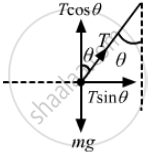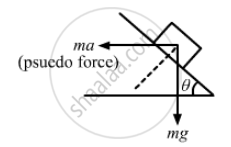Department of Pre-University Education, KarnatakaPUC Karnataka Science Class 11

# A Car is Speeding up a Horizontal Road with Acceleration A. Consider the Following Situations in the Car: (I) a Ball Suspended - Physics

Sum

A car is speeding up a horizontal road with acceleration a. Consider the following situations in the car: (i) A ball suspended from the ceiling by a string is maintaining a constant angle with the vertical. Find this angle. (ii) A block is kept on a smooth incline and does not slip on the incline. Find the angle of the incline with the horizontal.

#### Solution

Let the pendulum (formed by the ball and the string) make angle θ with the vertical.From the free-body diagram,
Tcosθ − mg = 0
Tcosθ = mg
$\Rightarrow T = \frac{mg}{\cos \theta} . . . \left( i \right)$
And, ma − T sin θ = 0
⇒ ma = T sin θ
$\Rightarrow T = \frac{ma}{\sin \theta} . . . . \left( ii \right)$
$\Rightarrow \tan \theta = \frac{a}{g}$
$\Rightarrow \theta = \tan^{- 1} \frac{a}{g}$
So, the angle formed by the ball with the vertical is $\tan^{- 1} \left( \frac{a}{g} \right)$
(ii) Let the angle of the incline be θ.
From the diagram,⇒ ma cos θ = mg sin θ

$\frac{\sin \theta}{\cos \theta} = \frac{a}{g}$

$\tan \theta = \frac{a}{g}\theta = \tan^{- 1} \left( \frac{a}{g} \right)$
So, the angle of incline is $\tan^{- 1} \left( \frac{a}{g} \right)$

Concept: Newton's Third Law of Motion
Is there an error in this question or solution?

#### APPEARS IN

HC Verma Class 11, Class 12 Concepts of Physics Vol. 1
Chapter 5 Newton's Laws of Motion
Q 41 | Page 83